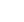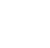# Probability and Statistics III: A Gentle Introduction to Statistics

## Description

This course provides an introduction to basic statistical concepts. We begin by walking through a library of probability distributions, where we motivate their uses and go over their fundamental properties. These distributions include such important folks as the Bernoulli, binomial, geometric, Poisson, uniform, exponential, and normal distributions, just to name a few. Particular attention is paid to the normal distribution, because it leads to the Central Limit Theorem (the most-important mathematical result in the universe, actually), which enables us to make probability calculations for arbitrary averages and sums of random variables. We then discuss elementary descriptive statistics and estimation methods, including unbiased estimation, maximum likelihood estimation, and the method of moments – you gotta love your MoM! Finally, we describe the t, χ2, and F sampling distributions, which will prove to be useful in upcoming statistical applications.

## Specific details

Category of Education Physical Science

## University

Georgia Institute of Technology

There are no comments posted here yetinfo@educom.net
 About Terms and ConditionsPrivacy PolicyRankingsAccreditations